# Grasp R Programming with Free Open-Source Books

### R Books

The R language (and open-source software) is the de facto standard among statisticians for the development of statistical software, and is widely used for statistical software development and data analysis. R is a modern implementation of S, one of several statistical programming languages designed at Bell Laboratories.

R is much more than a programming language. It’s an interactive environment for performing statistics. R offers a wide variety of statistical (linear and nonlinear modelling, classical statistical tests, time-series analysis, classification, clustering, …) and graphical techniques, and is highly extensible. The ability to download and install R packages is a key factor which makes R an excellent language to learn. Packages are the fundamental units of reproducible R code. They include reusable R functions, the documentation that describes how to use them, and sample data. The CRAN package repository hosts over 10,000 packages, and Bioconductor hosts nearly 1,300 packages.

R is available as Free Software under the terms of the Free Software Foundation’s GNU General Public License in source code form.

We have published a series covering the best open source programming books for other popular languages. Read them here.### An Introduction To R: Notes on R: A Programming Environment for Data Analysis and Graphics

By William N Venables, David M Smith, and the R Core Team (105 pages)

This tutorial manual provides a comprehensive introduction to R, a software package for statistical computing and graphics.

R supports a wide range of statistical techniques and is easily extensible via user-defined functions. One of R’s strengths is the ease with which publication-quality plots can be produced in a wide variety of formats.

Chapters explore:

• Simple manipulations; numbers and vectors
• Objects, their modes and attributes
• Ordered and unordered functions
• Arrays and matrices
• Lists and data frames
• Probability distributions
• Grouping, loops and conditional execution
• Statistical models in R
• Graphical procedures
• Packages

The manual is released under an open source license.

An Introduction to R is one of the R Manuals. Visit the Comprehensive R Archive Network to read the others.### R for Data Science: Import, Tidy, Transform, Visualize, and Model Data

By Hadley Wickham and Garrett Grolemund (522 pages)

R for Data Science teaches you how to do data science with R. It introduces the reader to R, RStudio, and the tidyverse, a collection of R packages designed to work together to make data science fast, fluent, and fun. Suitable for readers with no previous programming experience, R for Data Science is designed to get you doing data science as quickly as possible.

Learn how to:

• Explore – examine your data, generate hypotheses, and quickly test them. Dive into visualisation, learning the basic structure of a ggplot2 plot, and powerful techniques for turning data into plots. Learn the key verbs that allow you to select important variables, filter out key observations, create new variables, and compute summaries. Combine visualisation and transformation with your curiosity and skepticism to ask and answer interesting questions about data
• Wrangle – transform your datasets into a form convenient for visualisation and modelling
• Program – learn powerful R tools for solving data problems with greater clarity and ease. Learn skills that allow you to both tackle new programs and to solve existing problems
• Model – provide a low-dimensional summary that captures true “signals” in your dataset
• Communicate – learn R Markdown for integrating prose, code, and results, learn how to take exploratory graphics and turn them into expository graphics. R Markdown formats and workflow are covered### Text Mining with R

By Julia Silge and David Robinson (HTML)

This book serves as an introduction to text mining using the tidytext package and other tidy tools in R. The authors of this book developed the tidytext package.

The functions provided by the tidytext package are relatively simple; what is important are the possible applications. This book provides compelling examples of real text mining problems. The chapters cover:

• Tidy text format and the unnest_tokens() function. It also introduces the gutenbergr and janeaustenr packages
• Perform sentiment analysis on a tidy text dataset, using the sentiments dataset from tidytext and inner_join() from dplyr
• Describes the tf-idf statistic (term frequency times inverse document frequency)
• Introduces n-grams and how to analyze word networks in text using the widyr and ggraph packages
• Methods for tidying document-term matrices and corpus objects from the tm and quanteda packages, as well as for casting tidy text datasets into those formats
• Explores the concept of topic modeling, and uses the tidy() method to interpret and visualize the output of the topicmodels package
• Case studies### The R Inferno

By Patrick Burns (126 pages)

The R Inferno is an essential guide to the trouble spots and oddities of R. The book shares a lot of useful information and maintains the reader’s interest. The book provides many useful techniques and tips for reducing memory usage, improving performance, and avoiding errors in computational analysis.

R is regarded as an excellent computing environment for most data analysis tasks. R is free, released under an open-source license, and has thousands of contributed packages. It is used in such diverse fields as ecology, finance, genomics and music.

• Falling into the Floating Trap
• Growing Objects
• Failing to Vectorize – includes coverage on subscripting (a key part of effective vectorization), vecorized if, and looks at when vectorization is not possible
• Over-Vectorizing
• Not Writing Functions – the power of language is abstraction. To make abstractions in R the programmer writes functions. This chapter also highlights the importance of making functions as simple as possible
• Doing Global Assignment – which can be useful in memoization
• Tripping on Object Orientation – S3 methods (including generic functions, the methods function, and inheritance) S4 methods (multiple dispatch, S4 structure), and Namespaces
• Believing It Does as Intended – looks at ghosts, chimeras, and devils – exorcised using the browser function
• Seeking Help

The book is illuminated with famous Botticelli artwork: The Giants, The Sowers of Discord, and The Thieves.

Note: The book is not officially released under an open source license, but Patrick Burns seems fine about it being treated as open.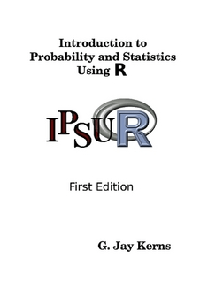### Introduction to Probability and Statistics Using R

By G. Jay Kerns (412 pages)

Introduction to Probability and Statistics Using R is a textbook for an undergraduate course in probability and statistics. The approximate prerequisites are two or three semesters of calculus and some linear algebra. Students attending the class include mathematics, engineering, and computer science majors.

Chapters cover:

• An Introduction to Probability and Statistics
• An Introduction to R: Installation, Basic R Operations and Concepts, Assignment, Object names, and Data types, Vectors
• Data Description: Introduces the different types of data that a statistician is likely to encounter
• Probability: Defines the basic terminology associated with probability and derive some of its properties, discusses three interpretations of probability, conditional probability and independent events, along with Bayes’ Theorem. The chapter concludes with an introduction to random variables
• Discrete Distributions: Introduces discrete random variables, discusses probability mass functions and some special expectations, namely, the mean, variance and standard deviation. Important discrete distributions are examined in detail, and attention is given to the concept of expectation and the empirical distribution
• Continuous Distributions: Continuous random variables and the associated PDFs and CDFs. The continuous uniform distribution is highlighted, along with the Gaussian, or normal, distribution. Some mathematical details pave the way for a catalogue of models
• Multivariate Distributions: Studies the notion of dependence between random variables in some detail
• Sampling Distributions: The bridge from probability and descriptive statistics
• Estimation: Discusses two branches of estimation procedures: point estimation and interval estimation
• Hypothesis Testing: Tests for Proportions, One Sample Tests for Means and Variances, Two-Sample Tests for Means and Variances, Other Hypothesis Tests, Analysis of Variance, Sample Size and Power
• Simple Linear Regression: Estimation, Model Utility and Inference, Residual Analysis, and Other Diagnostic Tools
• Multiple Linear Regression: The Multiple Linear Regression Model, Estimation and Prediction, Model Utility and Inference, Polynomial Regression, Interaction, Qualitative Explanatory Variables, Partial F Statistic, Residual Analysis and Diagnostic Tools
• Resampling Methods: Bootstrap Standard Errors, Bootstrap Confidence Intervals, Resampling in Hypothesis Tests
• Categorical Data Analysis
• Nonparametric Statistics
• Time Series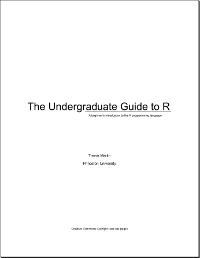### The Undergraduate Guide to R

By Trevor Martin (68 pages)

The Undergraduate Guide to R is a beginner’s introduction to the R programming language.

After reading this book, you’ll be able to perform most common data manipulating, analyzing, comparing and viewing tasks with R. The book also provides the necessary foundation blocks to enable the reader to progress to more advanced R techniques, and offers general tips and suggestions about how to code in R.

The Undergraduate Guide to R is written so that the reader needs no prior knowledge of programming (although basic knowledge of general computer skills and statistics is essential).

Sections cover:

• What is R?
• How to Install R
• The Basics: Algebra, Vectors, Matrices, Manipulation to arrange your data, and Loops/Statements (for-loop, if-statement, ifelse-statement)
• Data Types: Types, Converting/Using
• Reading in Data: Types of Data, How to Read In Data
• Plotting Data: Dot Plots, Histograms, Box Plots, and Additions
• Exporting Data: Types of Output, How to Export Data
• Functions: Built In, Custom
• Tips for Writing Good R Code: General, Matrix Multiplication, Plan, Debug, Help, Packages
• R Editors: Besides the RGui built-in editor, this chapter gives links to other popular editors for R, including WinEDT, Tinn-R, and explains that other popular editors such as Eclipse and Emacs can be configured to use R syntax highlighting

The book is freely available, licensed under Creative Commons Attribution-NonCommercial-NoDerivs 3.0 Unported (CC BY-NC-ND 3.0).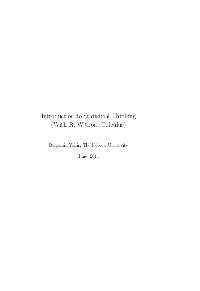### Introduction to Statistical Thinking (With R, Without Calculus)

By Benjamin Yakir (324 pages)

Introduction to Statistical Thinking is targeted at college students who need to learn statistics, students with little background in mathematics and often no motivation to learn more. This book uses the basic structure of generic introduction to statistics course.

Chapters cover:

• Short introduction to statistics and probability
• Data structures and variation
• Provides numerical and graphical tools for presenting and summarizing the distribution of data
• Fundamentals of probability: Concept of a random variable, Examples of special types of random variables, Normal random variable, Sampling distribution and presents the Central Limit Theorem and the Law of Large Numbers
• Discussion of statistical inference. It provides an overview of the topics that are presented in the subsequent chapter
• Basic tools of statistical inference, namely point estimation, estimation with a confidence interval, and the testing of statistical hypothesis
• Discusses inference that involve the comparison of two measurements
• Analysis of two case studies. The case studies apply the tools presented in the book

Much of the book is based on material from the online book “Collaborative Statistics” by Barbara Illowsky and Susan Dean.### ModernDive: An Introduction to Statistical and Data Sciences via R

By Chester Ismay and Albert Y. Kim (HTML)

ModernDive is a textbook that teaches students how to:

1. use R to explore and visualize data
2. use randomization and simulation to build inferential ideas
3. effectively create stories using these ideas to convey information to a lay audience

The book uses many R packages, and makes effective use real-world data sets to communicate key concepts. The book offers good treatment of the basics of data analysis (data wrangling and data exploration and data visualization, including the elegant roadmap for selecting a chart type shown below) and statistical concepts including simulation, regression and hypothesis testing.

The book also aims to give students an understanding of the overarching data analysis process, including concepts like reproducibility and telling stories with data.

This book is written using the CC0 1.0 Universal License.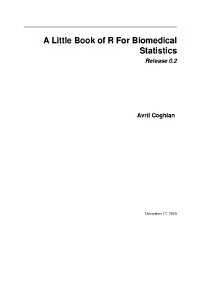### A Little Book of R for Biomedical Statistics

By Avril Coghlan (35 pages)

Little Book of R for Biomedical Statistics is a simple introduction to biomedical statistics using the R statistics software.

This booklet tells you how to use the R software to carry out some simple analyses that are common in biomedical statistics. In particular, the focus is on cohort and case-control studies that aim to test whether particular factors are associated with disease, randomised trials, and meta-analysis.

This booklet assumes the reader has some basic knowledge of biomedical statistics, and the principal focus of the booklet is not to explain biomedical statistics analyses, but instead to explain how to carry out these analyses using R.

The booklet examines:

• Calculating Relative Risks for a Cohort Study
• Calculating Odds Ratios for a Cohort or Case-Control Study
• Testing for an Association Between Disease and Exposure, in a Cohort or Case-Control Study
• Calculating the (Mantel-Haenszel) Odds Ratio when there is a Stratifying Variable
• Testing for an Association Between Exposure and Disease in a Matched Case-Control Study
• Dose-response analysis
• Calculating the Sample Size Required for a Randomised Control Trial
• Calculating the Power of a Randomised Control Trial
• Making a Forest Plot for a Meta-analysis of Several Different Randomised Control Trials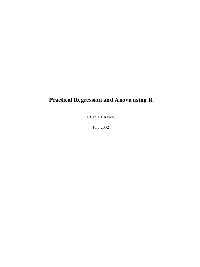### Practical Regression and Anova in R

By Julian J. Faraway (213 pages)

Practical Regression and Anova in R is an intermediate text on the practice of regression and analysis of variance. The objective is to learn what methods are available and more importantly, when they should be applied. The book is not an introduction to R.

Chapters cover:

• Estimation
• Inference
• Errors in Predictors
• Generalized Least Squares
• Testing for Lack of Fit
• Diagnostics
• Transformation
• Scale Changes, Principal Components and Collinearity
• Variable Selection
• Statistical Strategy and Model Uncertainty
• Chicago Insurance Redlining – a complete example
• Robust and Resistant Regression
• Missing Data
• Analysis of Covariance
• ANOVA (Analysis of Variance)

Permission to reproduce individual copies of this book for personal use is granted.

• Learning Statistics with R – covers the contents of an introductory statistics class, as typically taught to undergraduate psychology students, focusing on the use of the R statistical software.

The license terms of these books are unclear.

#### Ada, Assembly, Awk, Bash, C, C++, C#, Clojure, CoffeeScript, ECMAScript, Erlang, Forth, Fortran, Go, Haskell, HTML, Java, JavaScript, LaTeX, Lisp, Logo, Lua, OCaml, Pascal, Perl, PHP, Prolog, Python, R, Ruby, Rust, Scala, Scheme, Scratch, SQL, Swift, TeX, VimL

•jryan14ify says: